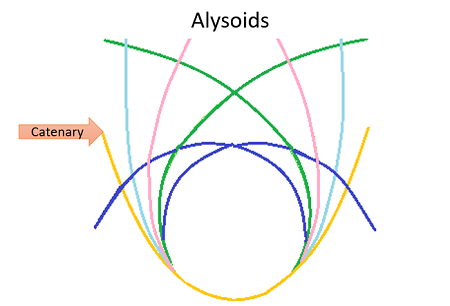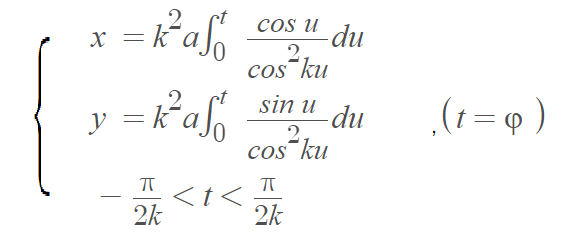# Alysoid definition, graph & formula

The alysoid (from the Greek Alusion, meaning “little chain”) is a transcendental curve first studied by Italian mathematician Ernesto Cesàro in 1886.The curve can be formed by a flexible chain of an infinite number of links, hanging by its own weight , but there are other ways to form the curve. More precisely, it is a curve where the center of curvature describes a parabola rolling over — and perpendicular to — a straight line.

The curve is sometimes called a catenary, which is actually a special case of the alysoid . In other words, the alysoid could more accurately be described as a generalization of the catenary.

## Equations for the Alysoid

1. aRc = s2 + b2; b ≠ 0.
2. s = b tan(kφ), k = b/a.

The form: s = tan kφ, where s is the arc length, is called the intrinsic Whewell equation.

Cartesian parameterization:## Relationship to some other curves

• The alysoid is similar to, but not the same as a catenary. The alysoid is a catenary if a = b (k = 1).
• When b is zero, the curve becomes a particular case of pseudo-spiral of Pirondini, called antiloga.

## References

 Schwartzman, S. (1994). The Words of Mathematics: An Etymological Dictionary of Mathematical Terms. Mathematical Association of America.
 Yates, R. Curves and their properties. National Council of Teachers of Mathematics, Washington D.S. Classics in Mathematics Education, Volume 4 (1906) from: https://citeseerx.ist.psu.edu/viewdoc/download?doi=10.1.1.853.7639&rep=rep1&type=pdf

CITE THIS AS:
Stephanie Glen. "Alysoid definition, graph & formula" From StatisticsHowTo.com: Elementary Statistics for the rest of us! https://www.statisticshowto.com/alysoid/